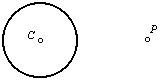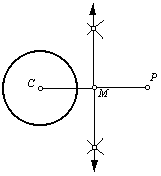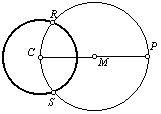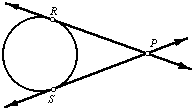Given a circle, its center point, and a point on the exterior of the circle, construct a line through the exterior point, tangent to the circle.

 1. Begin with a circle centered on point C. Point P is on the exterior of the circle.2. Draw line segment CP, and construct point M, the midpoint of line segment CP. (Refer to the perpendicular bisector construction for the construction of the midpoint.)3. Center the compass on point M. Draw a circle through points C and P. It will intersect the other circle at two points, R and S.4. Points R and S are the tangent points. Lines PR and PS are tangent to the circle centered on point C.Back to Geometry Construction Reference